# 2. 分析方法与过程

## 2.1 分析过程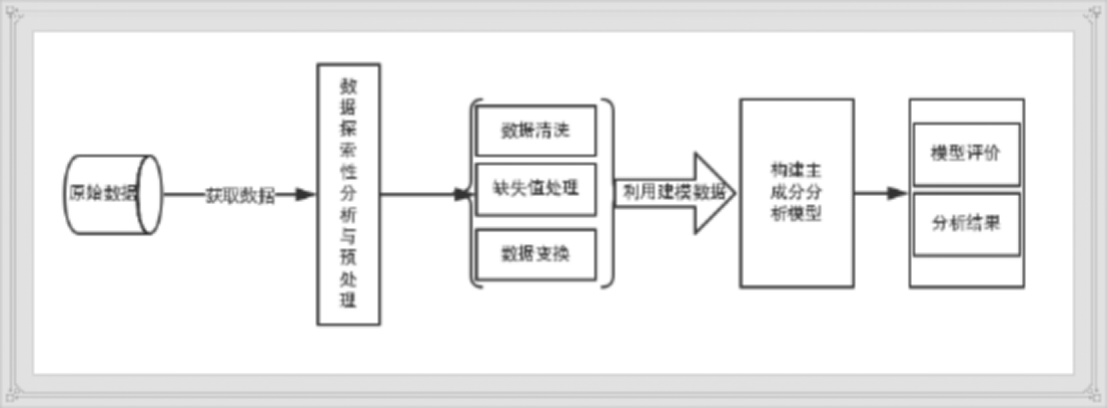1）从NBA中国官方网站获取原始数据；2)对样本数据进行探索性分析，剔除与球员实际价值没有关联的数据特征；3）对样本数据进行预处理，包括数据清洗、属性规约和数据变换；4）构建建模样本集；5)构建主成分分析模型；6）根据模型输出的结果进行分析。

# 3. 获取数据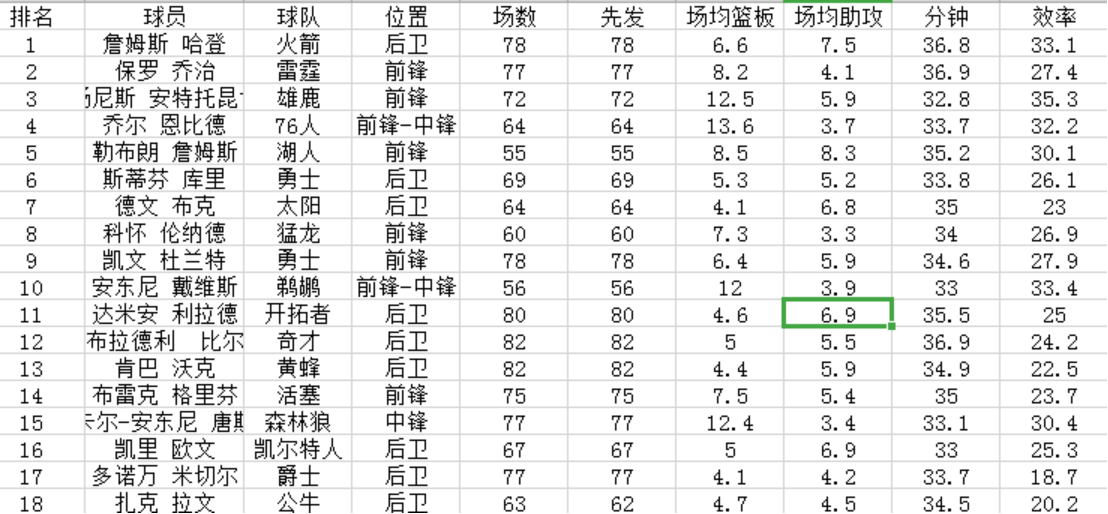# 4. 数据探索性分析与预处理

## 4.1探索性分析

### 4.1.1 条形图分析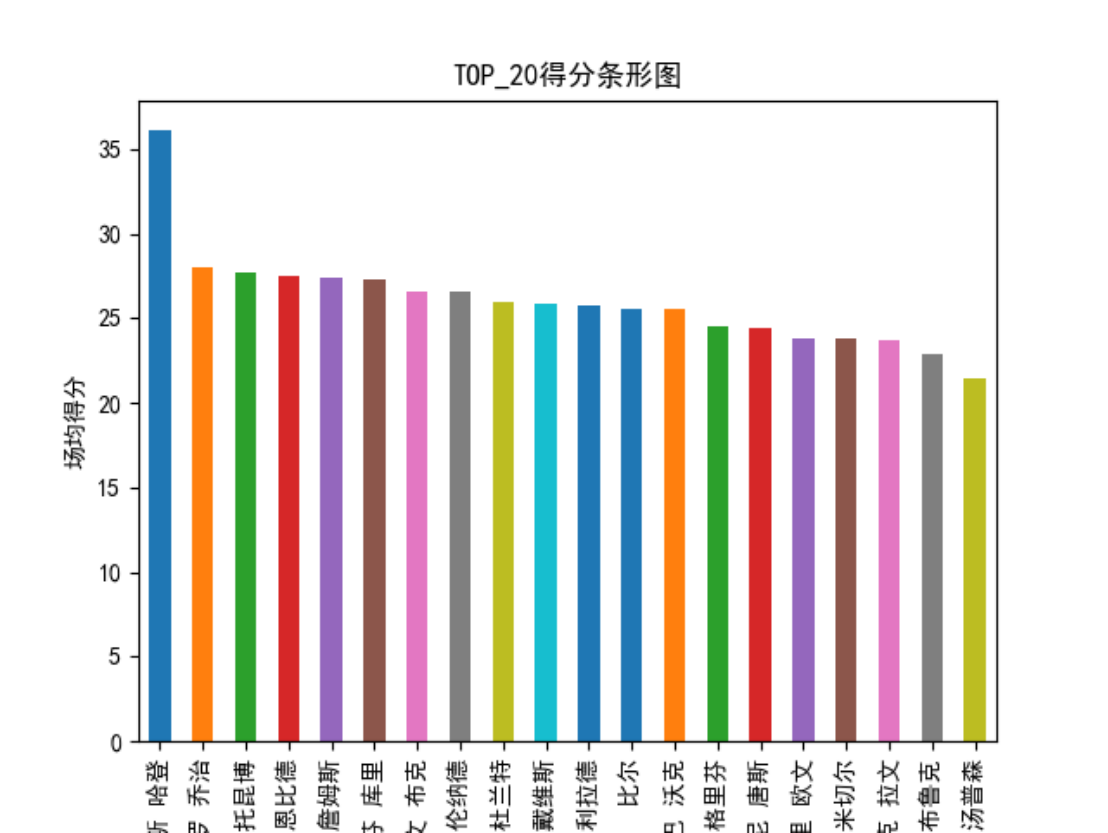### 4.1.2 散点图分析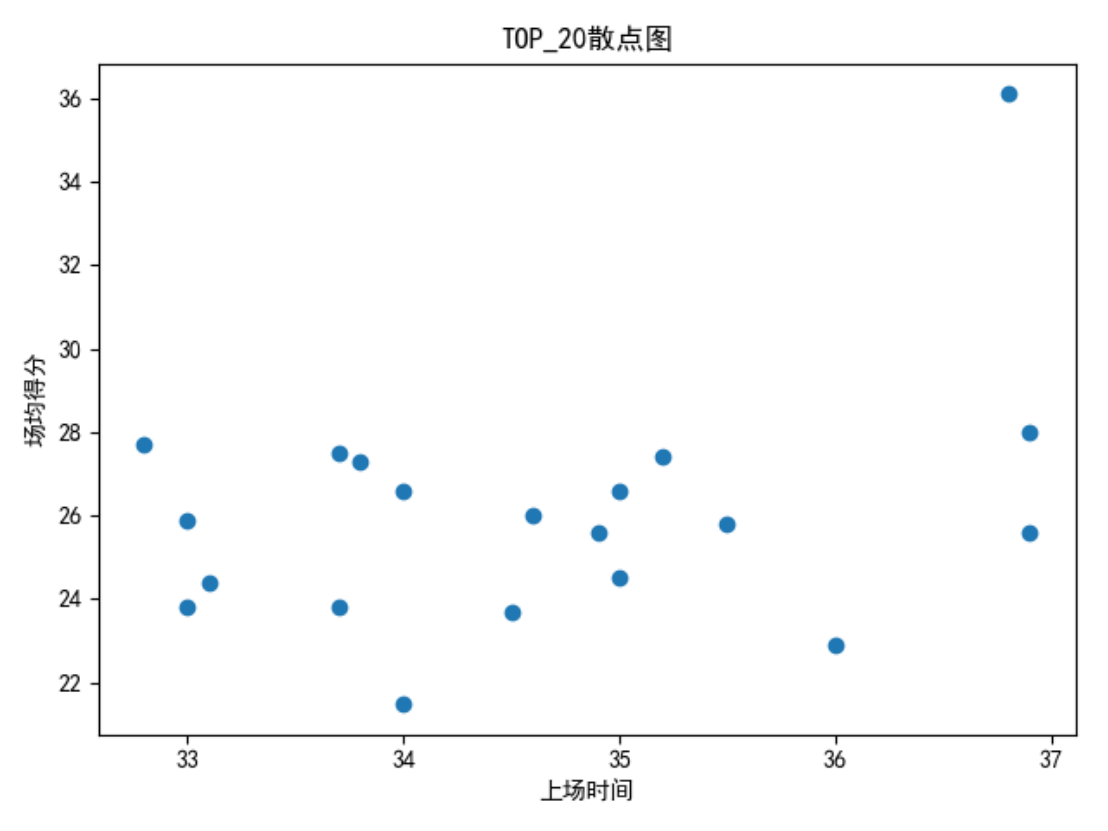## 4.2 数据预处理

### 4.2.1 数据清洗

1. 考虑到受伤和轮休球员的原因，将上场场次过滤掉。
2. 由于得分能力在前50的球员基本都是首发登场，所以过滤掉首发场次。
3. 球员的犯规次数与球员的实际价值没有太多关联，因此过滤掉。
4. 由于是主成分分析建模找出最高实际价值球员，那么只需要考虑场均得分前20的球员，其他球员过滤掉。

# 5. 构建主成分分析模型与结果分析

## 5.1 构建建模数据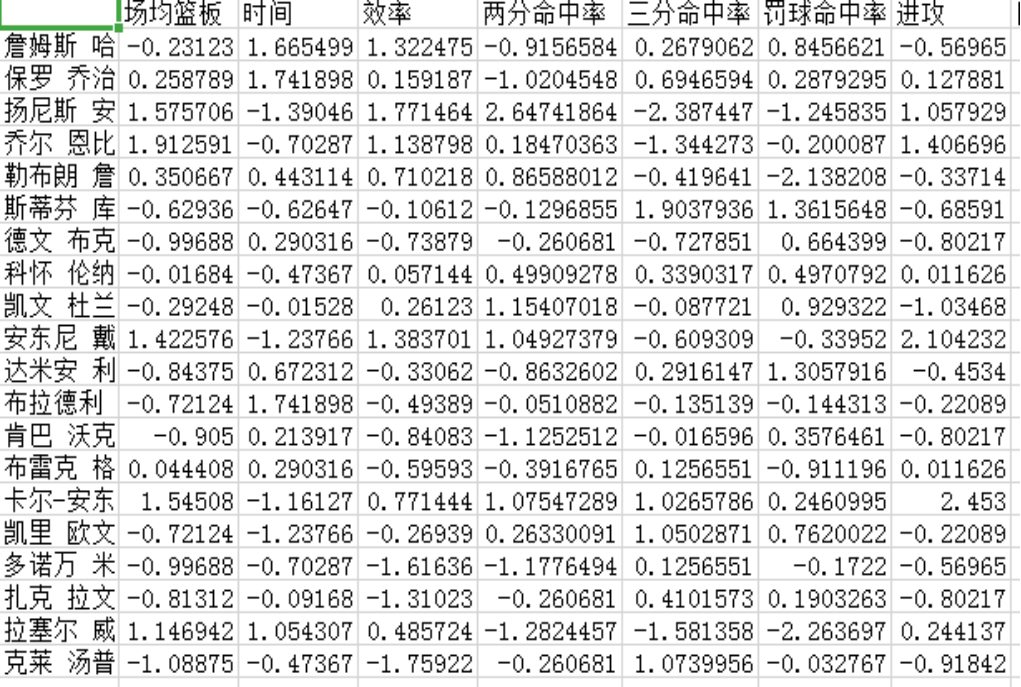## 5.2 构建模型与结果分析

### 5 .2.1 导入数据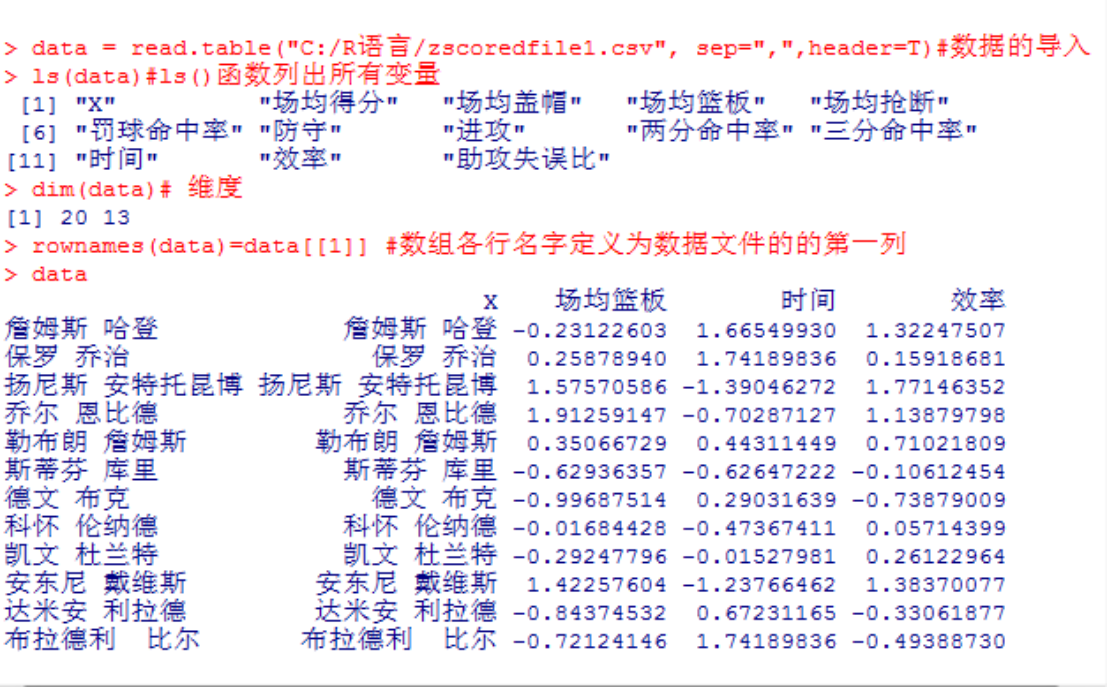### 5.2.2 计算相关系数矩阵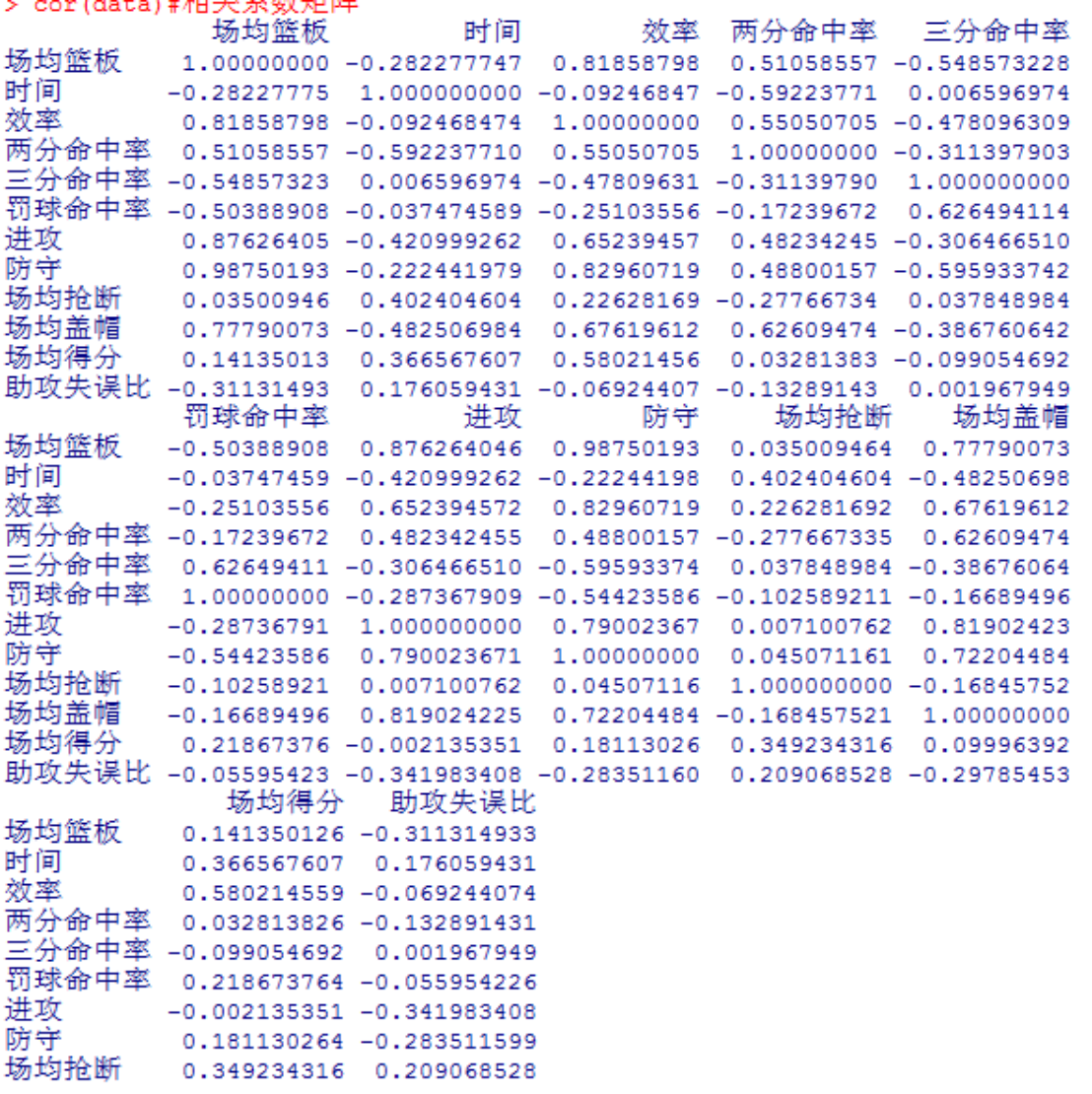### 5.2.3 PCA综合信息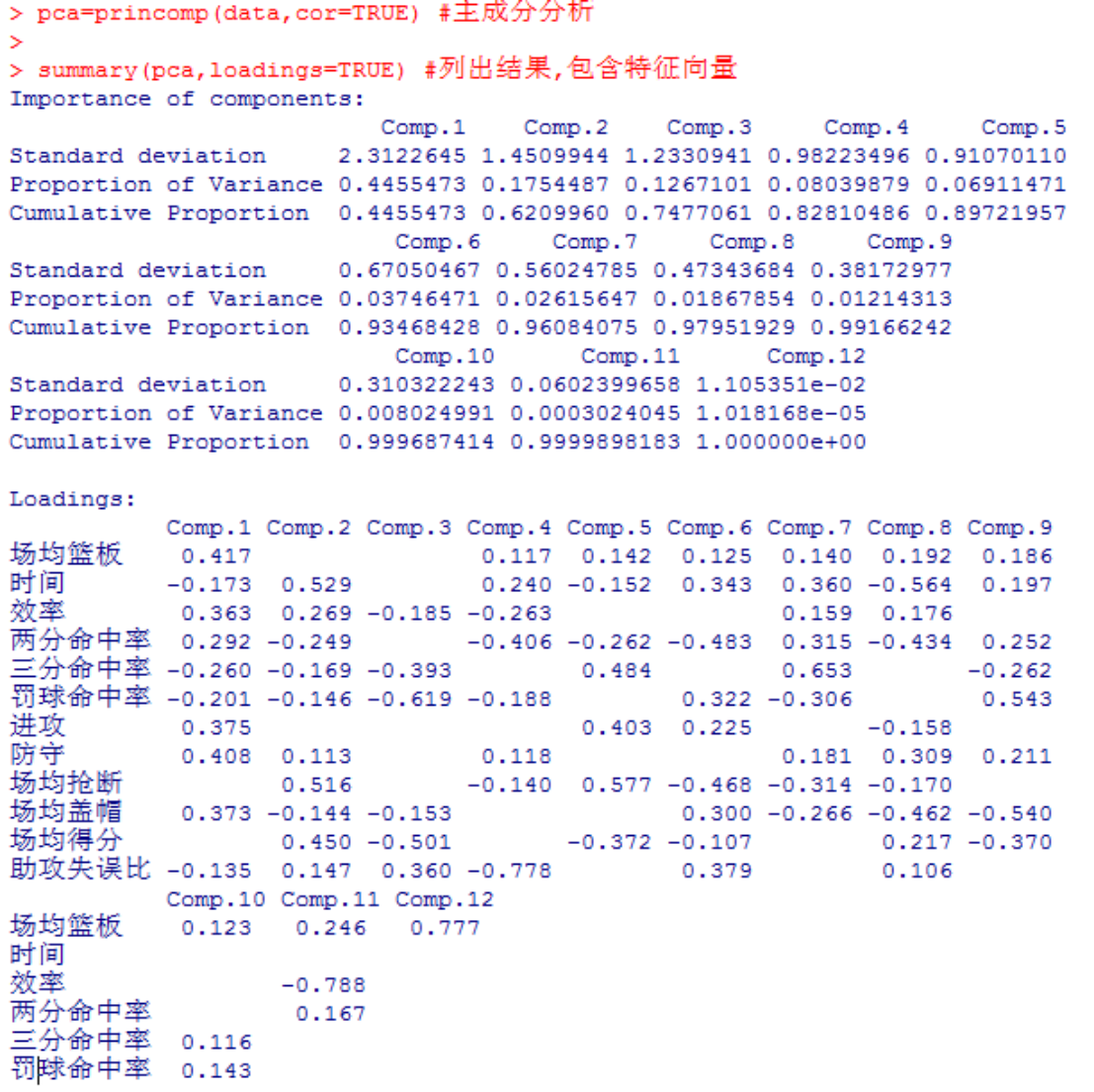### 5.2.4 确定主成分个数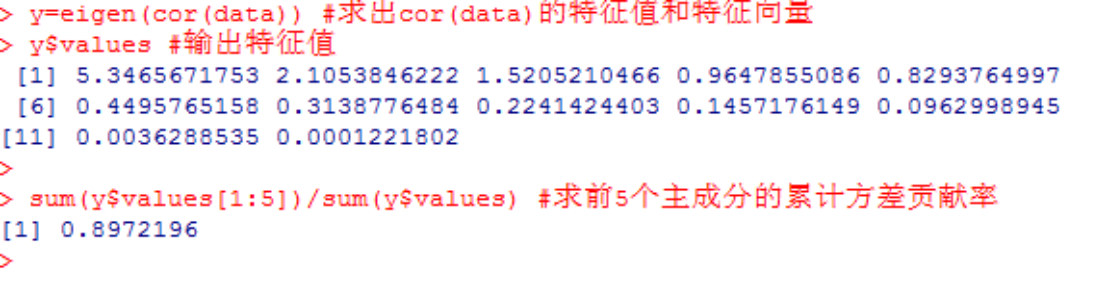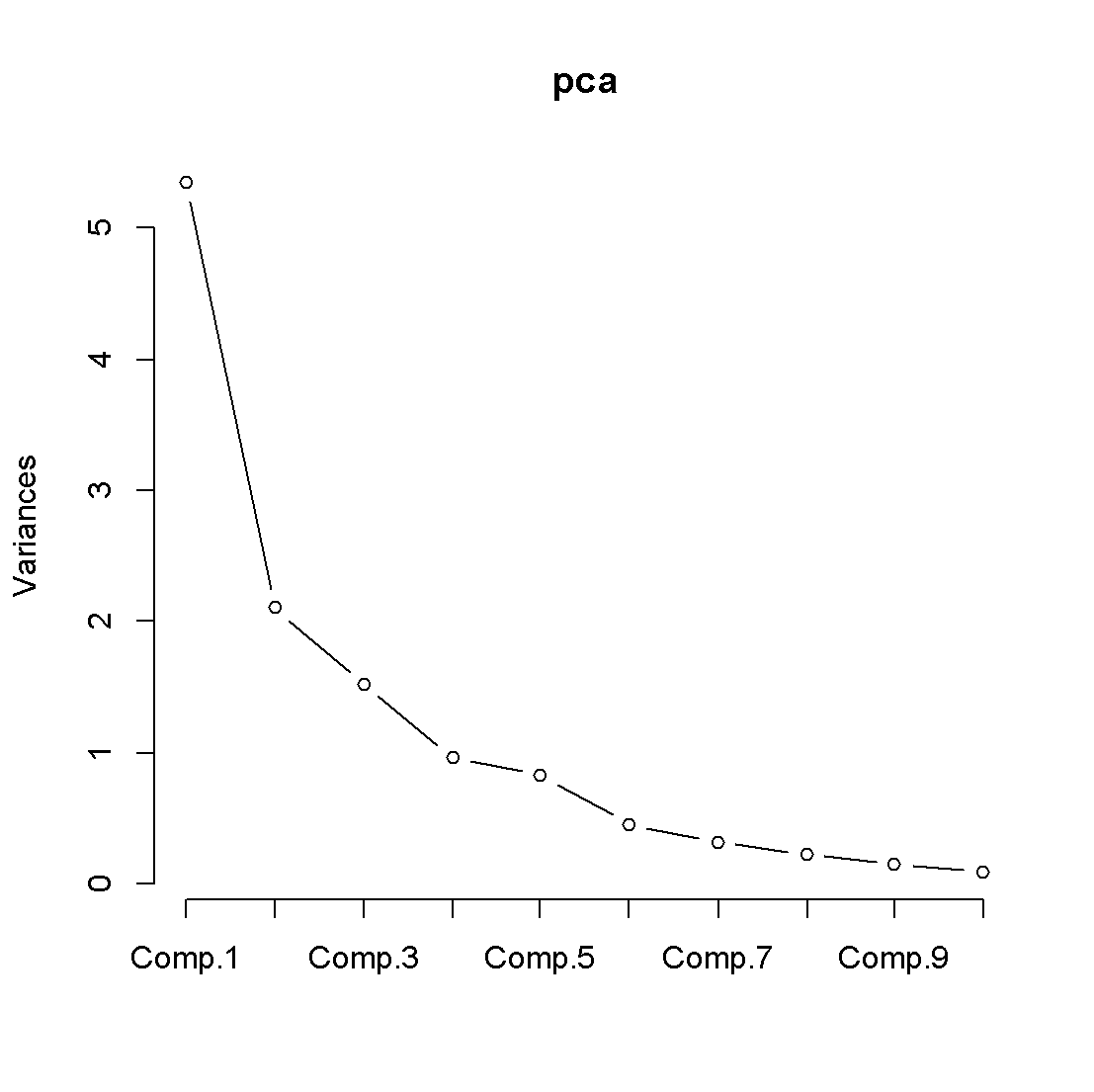### 5.2.5 主成分散点图分析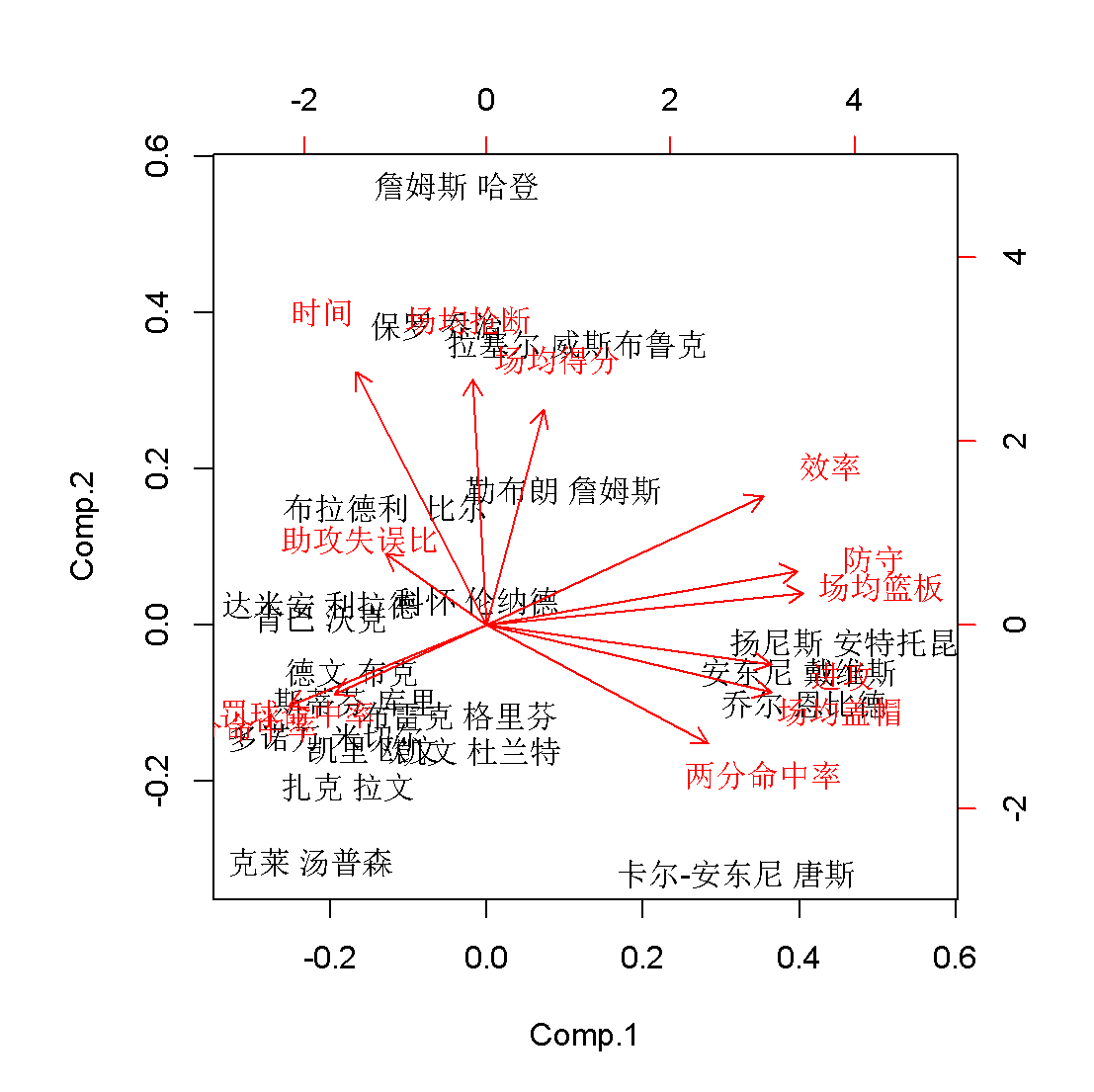### 5.2.6 主成分得分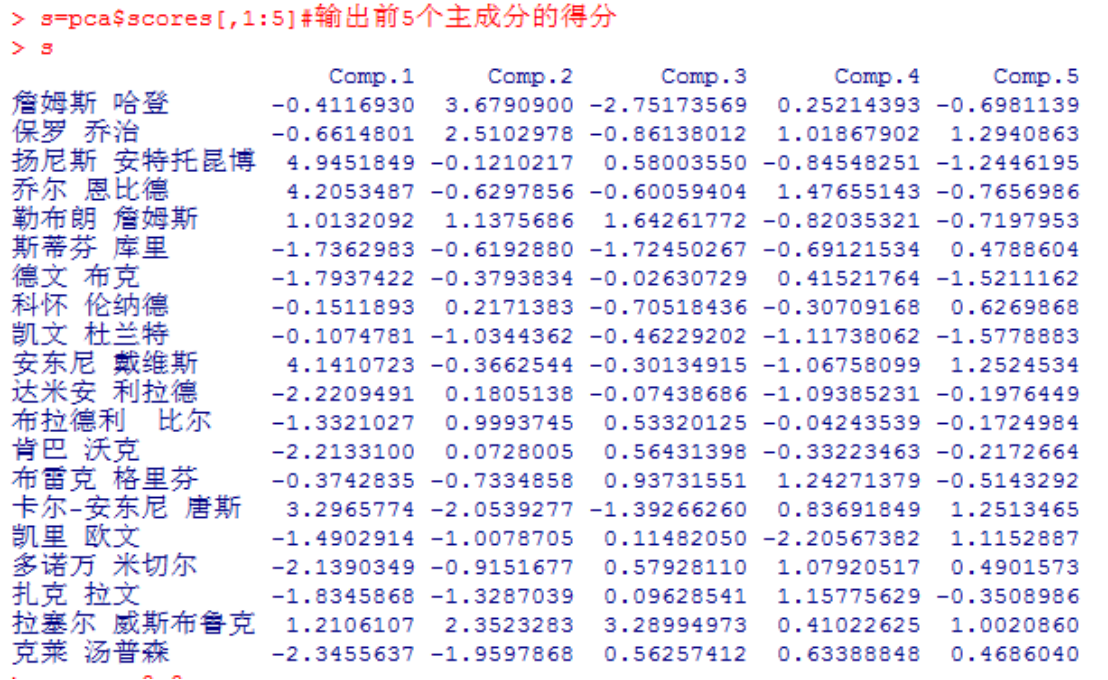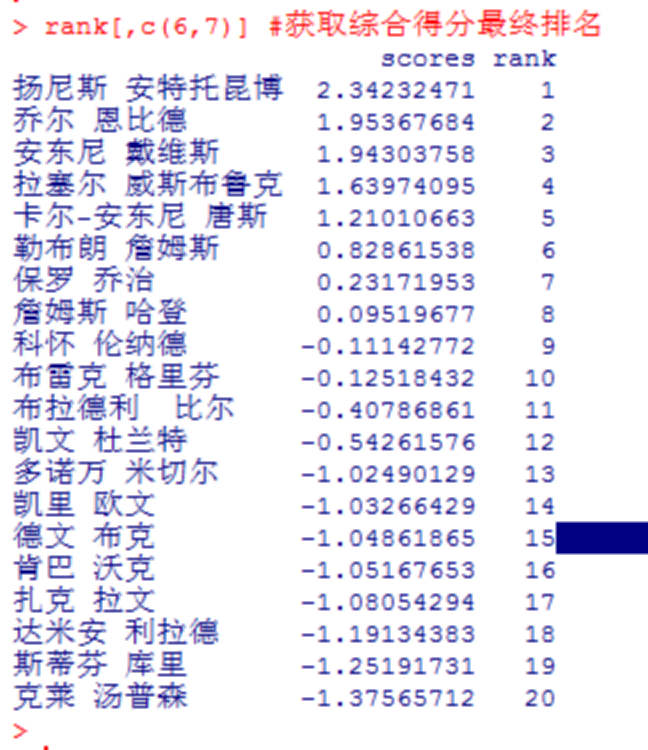# 6. 总结

1. 只采用了单一的方法进行建模，没有与之比较的方法。
2. 数据预处理过于简单，不够深入，可能无法真正的体现数据的内在价值。
3. 本文虽然得出的最具有实际价值的球员，但从最后的综合得分可以看出，本文所用数据建立的模型对于锋线球员有利，对于后卫线球员不利，库里是NBA联盟最近几年表现很好的球员，可此模型没有体现出库里这名球员的价值。所以模型有待改进。

# 附录

## 1. 爬虫代码如下

``````# -*- coding: utf-8 -*-
"""

"""

import json
import csv
import requests

url = 'https://china.nba.com/static/data/league/playerstats_All_All_All_0_All_false_2018_2_All_Team_points_All_perGame.json'
header = {'user-agent': 'Mozilla/5.0 (Macintosh; Intel Mac OS X 10_10_1)'
+ ' AppleWebKit/537.36 (KHTML, like Gecko) Chrome/37.0.2062.124 Safari/537.36'}
def get_page(url):
try:
if r.status_code == 200:
print(r.json())
return r.json()
except requests.ConnectionError as e:
print('Error', e.args)
def parse_page(json):
if json:
for item in items:
it = item['playerProfile']
its = item['teamProfile']
stat = item['statAverage']
player={}
player['排名'] = item['rank']
player['球员']=it['displayName']
player['球队'] = its['name']
player['位置'] = it['position']
player['场数'] = stat['games']
player['先发'] = stat['gamesStarted']
player['场均篮板'] = stat['rebsPg']
player['场均助攻'] = stat['assistsPg']
player['分钟'] = stat['minsPg']
player['效率'] = stat['efficiency']
player['2分命中率%'] = stat['fgpct']
player['3分命中率%'] = stat['tppct']
player['罚球命中率%'] = stat['ftpct']
player['进攻'] = stat['offRebsPg']
player['防守'] = stat['defRebsPg']
player['场均抢断'] = stat['stealsPg']
player['场均盖帽'] = stat['blocksPg']
player['失误'] = stat['turnoversPg']
player['犯规'] = stat['foulsPg']
player['场均得分'] = stat['pointsPg']
yield player

def write_tofile():
fieldnames = ['排名', '球员', '球队', '位置', '场数', '先发', '场均篮板',
'场均助攻', '分钟', '效率', '2分命中率%', '3分命中率%', '罚球命中率%',
'进攻', '防守', '场均抢断', '场均盖帽', '失误', '犯规', '场均得分']
with open('NBA_TOP50球员数据.csv', 'a', encoding='utf_8') as f:
writer = csv.writer(f)
writer.writerow(fieldnames)

for result in parse_page(get_page(url)):
# 输出json文件
# with open('NBA_TOP50球员数据.json', 'a', encoding='utf-8') as f:
#     f.write(json.dumps(result, indent=1, ensure_ascii=False))

# 输出csv文件
with open('NBA_TOP50球员数据.csv', 'a', encoding='utf-8') as f:
fieldnames = ['排名', '球员', '球队', '位置', '场数', '先发', '场均篮板',
'场均助攻', '分钟', '效率', '2分命中率%', '3分命中率%', '罚球命中率%',
'进攻', '防守', '场均抢断', '场均盖帽', '失误', '犯规', '场均得分']
writer = csv.DictWriter(f, fieldnames=fieldnames)
writer.writerow(result)

def main():
write_tofile()

if __name__ == '__main__':
main()

``````

## 2. 数据探索性分析及预处理代码如下

``````import pandas as pd

# 参数初始化
inputfile = 'NBA_TOP50球员数据.csv'
outputfile = 'zscoredfile1.csv' #标准化后的数据

data = pd.read_csv(inputfile, index_col='球员', engine='python', encoding='utf-8')
# 读入数据并以球员姓名作为行标签
# print(data.columns)  # 查看列名
data = data.iloc[:20]  # 获取前20行数据
# data.columns = ['X1', 'X2', 'X3', 'X4', 'X5', 'X6', 'X7', 'X8', 'X9', 'X10', 'X11', 'X12', 'X13', 'X14', 'X15']
data = data[['场均篮板', '场均助攻', '分钟', '效率', '2分命中率%', '3分命中率%',
'罚球命中率%', '进攻', '防守', '场均抢断', '场均盖帽', '失误', '犯规', '场均得分']]

new = data['场均助攻']/data['失误']  # 助攻失误比
new = pd.DataFrame(new)  # 转换成DataFrame结构数据
data = pd.concat([data, new], axis=1)  # 与之前的数据连接
data = data[['场均篮板', '分钟', '效率', '2分命中率%', '3分命中率%',
'罚球命中率%', '进攻', '防守', '场均抢断', '场均盖帽', '场均得分', 0]]
data.columns = ['场均篮板', '时间', '效率', '两分命中率', '三分命中率',
'罚球命中率', '进攻', '防守', '场均抢断', '场均盖帽', '场均得分', '助攻失误比']
print(data)

import matplotlib.pyplot as plt
plt.rcParams['font.sans-serif'] = ['SimHei'] #用来正常显示中文标签
plt.rcParams['axes.unicode_minus'] = False #用来正常显示负号

data['场均得分'].plot(kind='bar')
plt.ylabel('场均得分')
plt.xlabel('球员')
plt.title('TOP_20得分条形图')
plt.savefig('df.png')
plt.scatter(data['时间'], data['场均得分'])
plt.ylabel('场均得分')
plt.xlabel('上场时间')
plt.title('TOP_20散点图')
plt.show()``````

## 3. 主成分分析R语言代码如下

``````data = read.table("C:/R语言/zscoredfile1.csv", sep=",",header=T) #数据的导入
ls(data) #ls()函数列出所有变量
dim(data) #维度
rownames(data)=data[] #数组各行名字定义为数据文件的的第一列
data = data[,2:13]
data
class(data) #查看数据类型
cor(data) #相关系数矩阵
pca=princomp(data,cor=TRUE) #主成分分析
y=eigen(cor(data)) #求出cor(data)的特征值和特征向量
y\$values #输出特征值
sum(y\$values[1:5])/sum(y\$values) #求前5个主成分的累计方差贡献率
screeplot(pca,type='lines') #画出碎石图
biplot(pca) #画出主成分散点图
s=pca\$scores[,1:5] #输出前5个主成分的得分
s
scores=0.0
for (i in 1:5)
scores=(y\$values[i]*s[,i])/(sum(y\$values[1:5]))+scores
sort=cbind(s,scores) #输出综合得分信息
class(sort)
paiming=sort[order(sort[,6],decreasing=T),] #按最后一列降序排序
rank=c(1,2,3,4,5,6,7,8,9,10,11,12,13,14,15,16,17,18,19,20)
rank=cbind(paiming,rank)
rank[,c(6,7)] #获取综合得分最终排名``````